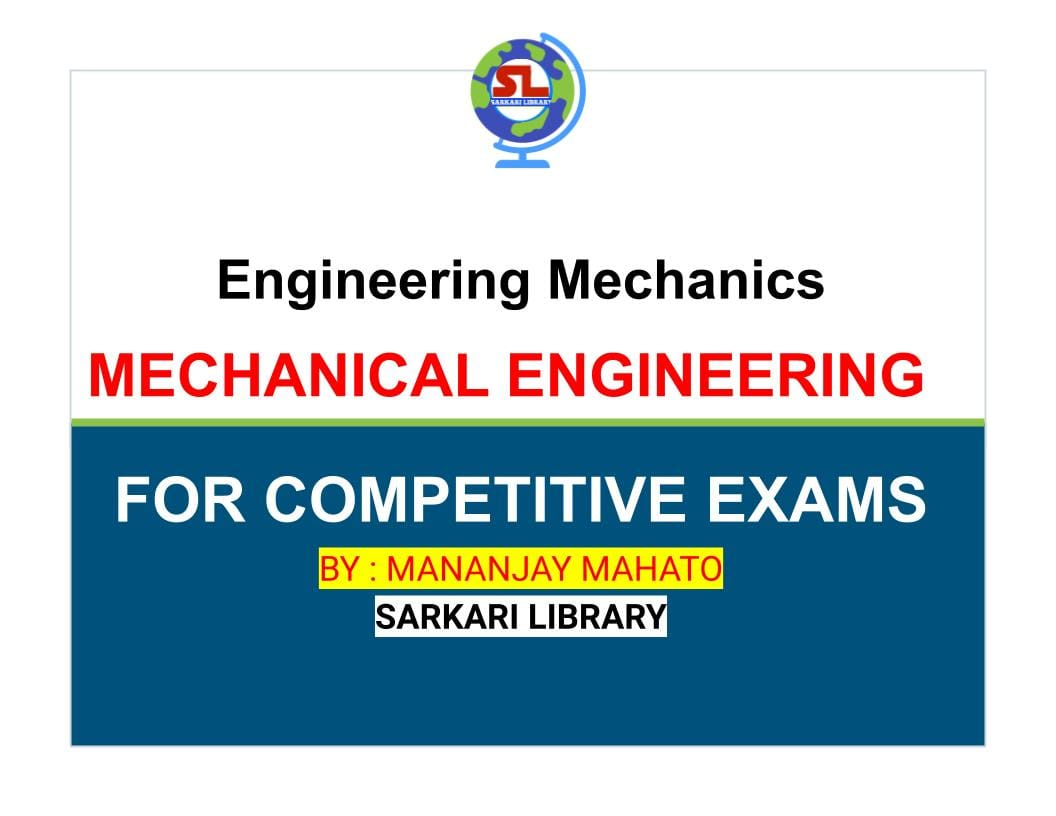## Engineering Mechanics : MECHANICAL ENGINEERING

• Post author:
• Post category:BlogEngineering Mechanics  : MECHANICAL ENGINEERING

# Engineering Mechanics

## MECHANICAL ENGINEERING

### BY: MANANJAY MAHATO

INTRODUCTION

Composition  and resolution of forces

Moment  and their applications

Parallel  forces and couples

Equilibrium  of Forces

Centre  of gravity

Moment  of inertia

Principle  of friction

Applications  of friction

Principles  of lifting machines

Simple  lifting machines

Support  reactions

Analysis  of perfect frames analytical method

Analysis  of perfect frames graphical method

Equilibrium  of Strings

Virtual  work

Linear  motion

Motion  under variable acceleration

Relative  velocity

Projectiles

Motion  of rotation

Combined  motion of rotation and translation

Simple  harmonic motion

Laws  of motion

Motion  of connected bodies

Helical springs and pendulum

Collision  of Elastic bodies

Motion  along a circular path

Balancing  of rotating masses

Work  power and energy

Kinetics of motion of rotation

Motion  of vehicles

Transmission  of power by belt and ropes

Transmission  of power by gear trains